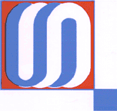IHEP 98 - 79
V.A. Maisheev
Gamma-Beam Propagation in the Anisotropic Medium
Protvino, 1998.- p.13, figs. 4, tables 1, refs.:18.

Propagation of $\gamma$-beam in the anisotropic medium is considered. The simplest example of this medium of a general type is a combination of the two linearly polarized monochromatic laser waves with different frequencies (dichromatic wave). The optical properties of this combination are described with the use of the permittivity tensor. The refractive indices and polarization characteristics of normal electromagnetic waves propagating in the anisotropic medium are found. The relations describing variations of $\gamma$-beam intensity and Stokes parameters as functions of the propagation length are obtained. The influence of laser wave intensity on the propagation process is calculated. The $\gamma$-beam intensity losses in the dichromatic wave depend on the initial circular polarization of $\gamma$-quanta. This effect also applies to single crystals which are oriented in some regions of coherent pair production. In principle, the single crystal sensitivity to circular polarization can be used for determination of polarization of high energy ( in tens GeV and more) $\gamma$-quanta and electrons.ZIPped PostScript PDF